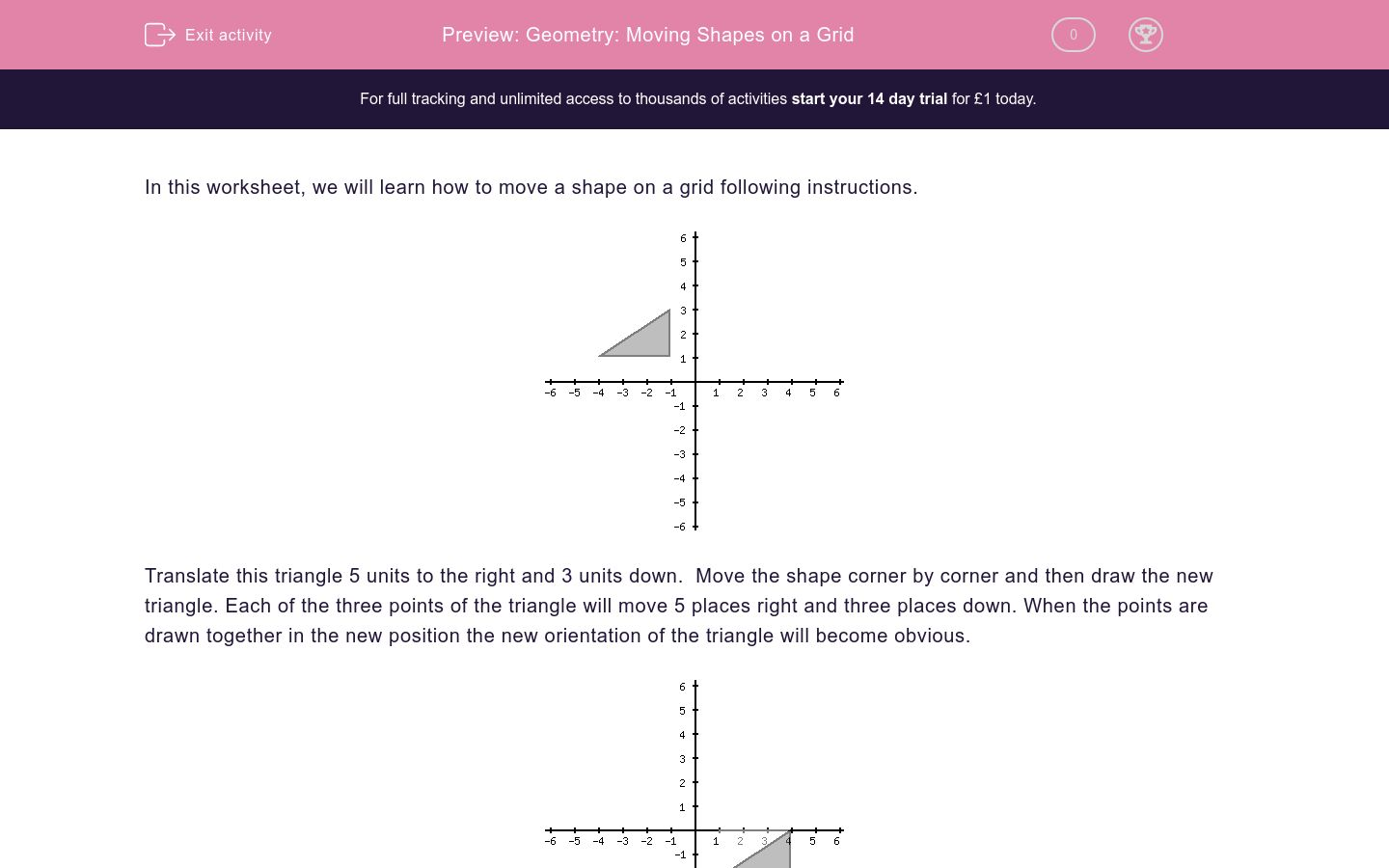# Geometry: Moving Shapes on a Grid

In this worksheet, students build their understanding of simple translation of shapes on a grid by following simple instructions.Key stage:  KS 2

Curriculum topic:   Maths and Numerical Reasoning

Curriculum subtopic:   Transformations

Difficulty level:### QUESTION 1 of 10

In this worksheet, we will learn how to move a shape on a grid following instructions.Translate this triangle 5 units to the right and 3 units down.  Move the shape corner by corner and then draw the new triangle. Each of the three points of the triangle will move 5 places right and three places down. When the points are drawn together in the new position the new orientation of the triangle will become obvious.The triangle is translated by moving a units to the right and b units down. Compare the old position with the new position. The number of units the new triangle has moved right is a. The number of units the the triangle has moved down is b.

Find the values of a and b?a=0 , b=2

a=2 , b=0

The triangle is translated by moving a units to the right and b units down. Compare the old position with the new position. The number of units the new triangle has moved right is a. The number of units the the triangle has moved down is b.

Find the values of a and b?a=0 , b=2

a=2 , b=0

The triangle is translated by moving a units to the right and b units down. Compare the old position with the new position. The number of units the new triangle has moved right is a. The number of units the the triangle has moved down is b.

Find the values of a and b?a=1 , b=4

a=4 , b=1

• Question 1

The triangle is translated by moving a units to the right and b units down. Compare the old position with the new position. The number of units the new triangle has moved right is a. The number of units the the triangle has moved down is b.

Find the values of a and b?a=0 , b=2
• Question 2

The triangle is translated by moving a units to the right and b units down. Compare the old position with the new position. The number of units the new triangle has moved right is a. The number of units the the triangle has moved down is b.

Find the values of a and b?a=2 , b=0
• Question 3

The triangle is translated by moving a units to the right and b units down. Compare the old position with the new position. The number of units the new triangle has moved right is a. The number of units the the triangle has moved down is b.

Find the values of a and b?a=4 , b=1
---- OR ----

Sign up for a £1 trial so you can track and measure your child's progress on this activity.

### What is EdPlace?

We're your National Curriculum aligned online education content provider helping each child succeed in English, maths and science from year 1 to GCSE. With an EdPlace account you’ll be able to track and measure progress, helping each child achieve their best. We build confidence and attainment by personalising each child’s learning at a level that suits them.

Get started